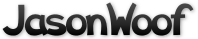Got questions, comments, patches, etc.? Contact Jason Woofenden
 author Jason Woofenden Sun, 22 May 2016 21:02:35 +0000 (17:02 -0400) committer Jason Woofenden Sun, 22 May 2016 21:08:18 +0000 (17:08 -0400)
Check return values of fscanf() (just bail early if it fails)

Don't use titles as scanf templates, compiler can't seem to figure out that
these come from string literals. Insteadd use fscanf(..., strlen...)

 score.c patch | blob | history

diff --git a/score.c b/score.c
index 8648679..8c5bc59 100644 (file)
--- a/score.c
+++ b/score.c
@@ -64,15 +64,21 @@ void
{
FILE *f;
{
FILE *f;
-       int i, j;
+       int i, j, ret;

f = open_score_file("r");
if(f) {
// If the file exists, read from it
for(j=0; j<2; j++) {

f = open_score_file("r");
if(f) {
// If the file exists, read from it
for(j=0; j<2; j++) {
-                       fscanf(f, titles[j]);
+                       ret = fseek(f, strlen(titles[j]), SEEK_CUR);
+                       if (ret != 0) {
+                               break;
+                       }
for(i = 0; i<N_SCORES; i++) {
for(i = 0; i<N_SCORES; i++) {
-                               fscanf(f, "%d %31[^\n]\n", &g_scores[j][i].score, g_scores[j][i].name);
+                               ret = fscanf(f, "%d %31[^\n]\n", &g_scores[j][i].score, g_scores[j][i].name);
+                               if (ret != 2) {
+                                       break;
+                               }
}
}
fclose(f);
}
}
fclose(f);
@@ -89,7 +95,7 @@ write_high_score_table()
if(f) {
// If the file exists, write to it
for(j=0; j<2; j++) {
if(f) {
// If the file exists, write to it
for(j=0; j<2; j++) {
-                       fprintf(f, titles[j]);
+                       fprintf(f, "%s", titles[j]);
for(i = 0; i<N_SCORES; i++) {
fprintf (f, "%d %.31s\n", g_scores[j][i].score, g_scores[j][i].name);
}
for(i = 0; i<N_SCORES; i++) {
fprintf (f, "%d %.31s\n", g_scores[j][i].score, g_scores[j][i].name);
}